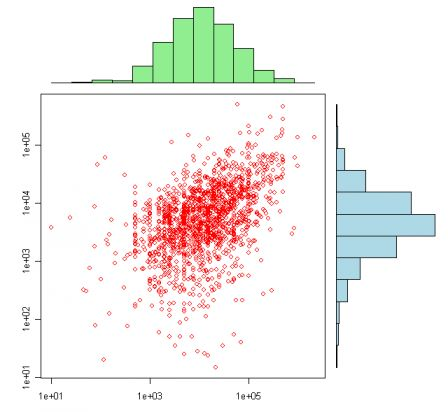Want to share your content on R-bloggers? click here if you have a blog, or here if you don't.

David Smith (alias @revodavid, see also on the Revolutions blog, here) pointed out that my R code was not easy to read (not only due to my computing skills, but mainly because of the typography I use). He suggested that I use the Pretty R tool (here). And I will…

So, just to answer quickly to a question I received by email (a few weeks ago, sorry for the delay), here is the code to get the following nice plot

```library(evd); data(lossalae)
x <- lossalae\$Loss; y <- lossalae\$ALAE
xhist <- hist(log(x), plot=FALSE)
yhist <- hist(log(y), plot=FALSE)
top <- max(c(xhist\$counts, yhist\$counts))
nf <- layout(matrix(c(2,0,1,3),2,2,byrow=TRUE),
c(3,1), c(1,3), TRUE)
par(mar=c(3,3,1,1))
plot(x, y, xlab="", ylab="",log="xy",col="red")
par(mar=c(0,3,1,1))
barplot(xhist\$counts, axes=FALSE, ylim=c(0, top),
space=0,col="light green")
par(mar=c(3,0,1,1))
barplot(yhist\$counts, axes=FALSE, xlim=c(0, top),
space=0, horiz=TRUE,col="light blue")```

I guess it is more convenient to read it, isn't it...